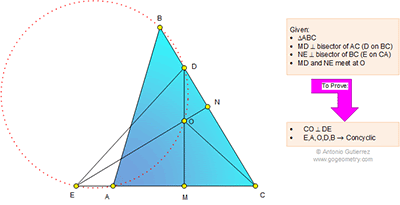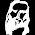## Monday, April 27, 2015

### Geometry Problem 1113: Triangle, Perpendicular Bisector, 90 Degrees, Circle, Concyclic Points, Cyclic Quadrilateral

Geometry Problem. Post your solution in the comments box below.
Level: Mathematics Education, High School, Honors Geometry, College.

Click the diagram below to enlarge it.#### 2 comments:

1.By construction, O is the orthocenter of ΔCDE, thus CO⊥DE.

∠BOA = 2∠C
∠BDA = 180° − 2∠CDA = 2∠C (since DA=DC)
Thus BDOA concyclic.

Also, (since EB=EC)
∠BEA = 180° − 2∠C
Thus BOAE concyclic.

Hence, EAODB concyclic.

2.Problem 1113
Is <MDC=<MDA=<ODA=90-<ACB=<AEO so EAOD is cyclic.
Is <OAB=90-<OAB/2=90-<ACB=<ODC so AODB is cyclic.
Therefore E,A,O,D and B are concyclic.
APOSTOLIS MANOLOUDIS 4 HIGH SCHOOL KORYDALLOS PIRAEUS GREECE